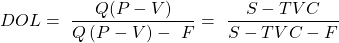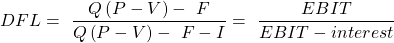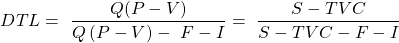Concept 63: Calculating Degree of Operating Leverage, Financial Leverage, and Total Leverage | IFT World
101 Concepts for the Level I Exam

# Concept 63: Calculating Degree of Operating Leverage, Financial Leverage, and Total LeverageDegree of operating leverage (DOL) measures operating risk. It is the ratio of the percentage change in operating income to the percentage change in quantity sold.where:

Q: Quantity sold,

P: Unit price,

V: Variable cost per unit,

F: Fixed operating costs

S: Dollar amount of sales,

TVC: total variable costs,

Degree of financial leverage (DFL) measures financial risk. It is the ratio of percentage change in net income to percentage change in operating income.where:

Q: Quantity sold,

P: Unit price,

V: Variable cost per unit,

F: Fixed costs

I: Fixed financial costs

Degree of total leverage (DTL) combines DOL and DFL. It is the ratio of percentage change in net income to percentage change in units sold.Crash Courses for November CFA Level I, II exams are coming soon!
This is default text for notification bar